# Legendre Polynomials

Also found in: Wikipedia.

## Legendre polynomials

[lə′zhän·drə ‚päl·i′nō·mē·əlz]
(mathematics)
A collection of orthogonal polynomials which provide solutions to the Legendre equation for nonnegative integral values of the parameter.
McGraw-Hill Dictionary of Scientific & Technical Terms, 6E, Copyright © 2003 by The McGraw-Hill Companies, Inc.
The following article is from The Great Soviet Encyclopedia (1979). It might be outdated or ideologically biased.

## Legendre Polynomials

a system of polynomials of successively increasing degree. The polynomials were first investigated by A. Legendre and P. Laplace independently of each other between 1782 and 1785. For n = 0, 1, 2, …, the Legendre polynomials Pn(x) can be defined by the formula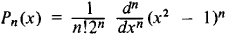The first few polynomials are

p0 (x) = 1

p1 (x) = x

p2 (x) = 1/2(3x2 - 1)

p3 (x) = 1/2(5x2 - 3x)

p4 (x) = 1/8(35x4 - 30x2 + 3)

p5 (x) = 1/8(63x5 - 70x3 + 15x)

All the zeros of P n (x) are real, lie in the interval [–1, + 1], and alternate with the zeros of Pn + 1 (x). The Legendre polynomials are a complete set of orthogonal polynomials on the interval [–1, + 1]. Thus, it is possible to expand an arbitrary function /(jc) integrable over the interval [– 1, +1] in a series of Legendre polynomials: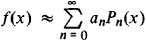where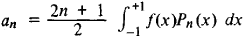The type of convergence of this series is roughly the same as that of a Fourier series. The Legendre polynomials are given explicitly by the formula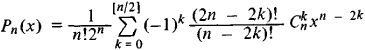The generating function is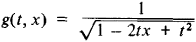that is, the Legendre polynomials are the coefficients in the expansion of this function in powers of t. They are recursively defined by

nPn (x) + (n - 1)Pn-2 (x) - (2n - 1)xPn-1 (x) = 0

Pn (x)satisfies the differential equation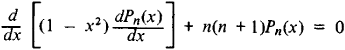which arises when separating the variables in Laplace’s equation in spherical coordinates.

### REFERENCES

Janke, E., F. Emde, and F. Lösch. Spetsial’nye funktsii; grafiki, tablitsy, 2nd ed. Moscow, 1968. (Translated from German.)
Lebedev, N. N. Spetsial’nye funktsii i ikh prilozheniia, 2nd ed. MoscowLeningrad, 1963.

V. I. BITIUTSKOV

The Great Soviet Encyclopedia, 3rd Edition (1970-1979). © 2010 The Gale Group, Inc. All rights reserved.
References in periodicals archive ?
In this analysis, the permanent environmental and genetic animal effects were modeled by Legendre polynomials of order two.
Alpert and Rokhlin  consider the special case of converting between Legendre polynomials and Chebyshev polynomials of first kind.
Furthermore, milk yields at peak lactation stages were shown to be high, for that reason, the 3rd order Legendre polynomials would not be the best fitting of the lactation curve.
n, and [P.sub.nk](x) ~ [x.sub.n], k = n, n + 1,....The sequence [P.sub.O] represents the shifted to the interval [0,1] Legendre polynomials; [P.sub.n], n > 0, is considered as an auxiliary sequence of incomplete polynomials, and the sequence [P.sub.n] is introduced here as the alternative Legendre polynomials (ALP).
Assume the animal has n records, and define Legendre polynomials for the range of [u, v] = [-1, 1], the j-th standardised age [a.sup.*.sub.j] can be computed as (Kirkpatrick et al., 1994):
For example, in the case of Legendre polynomials [kappa](s) = [square root of (1 - [s.sup.2]] [tau](s) = -2s and
The covariates of the fixed regression coefficient for parity effect are fifth-order Legendre polynomials , with the exponential term of the Wilmink function ([e.sup.-0.05t]) as a sixth-order covariate [15,16].
The aim of this study was to estimate covariance functions for additive genetic, direct and maternal permanent environmental effects on the growth from 1 to 270 days of age using random regression models on Legendre polynomials (LPs).
The Adomian decomposition technique is improved via Chebyshevpolynomials in [9,10], with Legendre polynomials  and with Laguerre polynomials .
Set the Legendre-Gauss points [mathematical expression not reproducible] as the zeros of the Legendre polynomials [L.sub.N+1](t).
where k =1, 2, ..., [??] = 2n - 1, n =1, 2, ..., [2.sup.k-1], m = 0, 1, ..., M - 1 is the degree of the Legendre polynomials and M is a fixed positive integer, and [P.sub.m](t) are the Legendre polynomials of degree m.

Site: Follow: Share:
Open / Close